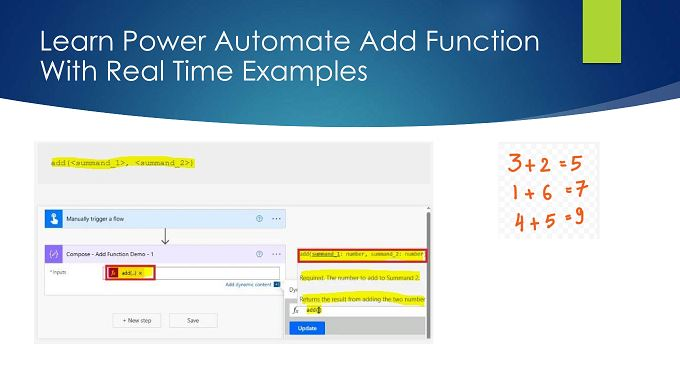# Power Automate Add Function: Real Time Examples 5 PlusIn this “Power Automate Add Function” article, we will learn about what an Add function is and how to work with the Add function in Power Automate Flow.

Power Automate, a part of Microsoft’s Power Platform, is a versatile tool for automating repetitive tasks and workflows. Among its many functions, the “Add” function stands out as a powerful tool for managing data and simplifying processes. In this article, we will explore the capabilities of Power Automate’s “Add” function with real-time examples to demonstrate how it can streamline your work and boost your productivity.

## What is the Add function in Power Automate?

The “Add” function is one of the Math functions in Power Automate which returns the result from adding two numbers. The “Add” function in Power Automate allows you to add data to various applications and services seamlessly. Whether you want to create new records in databases, add items to a SharePoint list, or append information to an Excel spreadsheet, this function is incredibly versatile.

### Syntax of Power Automate Add Function:

`add(<summand_1>, <summand_2>)`

Parameter:

 Parameter Required Type Description , Yes Integer, Float, or mixed The numbers to add

Output:

 Return value Type Description Integer or Float The result from adding the specified numbers

`add(2.5, 1.5)`

Output

It returns this result: 4

### Limitations in Power Automate Add Function

There is a limitation in terms of the length of the numbers we pass inside the add function. For example, if we try to perform the add between these two numbers, 12345678901234567891 and 1, we will get this error:

“The power flow’s logic app flow template was invalid. The template language expression ‘add(12345678901234567891,1)’ is not valid: the value ‘12345678901234567891’ at position ‘4’ cannot be converted to number.”

`add(12345678901234567891,1)`

Now, let’s perform the addition of these two numbers: 1234567890123456788 and 1.

`add(1234567890123456788,1)`

In this case, it won’t return any errors, but the sum-up output is not correct. This will give the output as “1234567890123456800” but the output should have been “1234567890123456789“.

From this example, we can say that even though the add function doesn’t result in an error, that does not mean the sum-up calculation is correct, so be careful while you pass numbers to the add function and validate the sum-up output.

## Power Automate Add Function Examples with Real-Time Scenario

In this section, we will see some of the real-time uses of the Add Function in Power Automate:

### Example 1: Calculate Monthly Expenses

Scenario: You want to use Power Automate to calculate your monthly expenses, given the expenses for the first two weeks of October.

```Set a variable: ExpenseWeek1
ExpenseWeek1 = 500

Set a variable: ExpenseWeek2
ExpenseWeek2 = 400

Set a variable: MonthlyExpense

Output:

The “Add” function will add the expenses for the first two weeks, and the MonthlyExpense variable will hold the result, which is \$900.

### Example 2: Calculate Total Sales

Scenario: In a sales report, you have the sales figures for Q3, and you want to calculate the total sales for that quarter.

```Set a variable: JulySales
JulySales = 15000

Set a variable: AugustSales
AugustSales = 18000

Set a variable: SeptemberSales
SeptemberSales = 22000

Set a variable: TotalQ3Sales

Output:

The “Add” function is used to add the sales figures for July, August, and September, and the TotalQ3Sales variable will hold the result, which is \$55,000.

### Example 3: Calculate Student Grades

Scenario: You have the scores of a student in two exams, and you want to calculate their total score.

```Set a variable: Exam1Score
Exam1Score = 85

Set a variable: Exam2Score
Exam2Score = 92

Set a variable: TotalScore

Output:

The “Add” function adds the scores of the two exams, and the TotalScore variable holds the result, which is 177.

### Example 4: Tracking Inventory

Scenario: You want to track the inventory of a product in a SharePoint list. Whenever new items are received, you want to update the current stock level in SharePoint.

• Create a SharePoint list named “ProductInventory” with columns: Product Name, Current Stock, and Received Quantity.
• Create a Power Automate flow triggered by new item arrivals.
• In your flow, use the “Get items” action to retrieve the current stock level for the product.
• Create a variable “ReceivedQuantity” to store the quantity of items received.
• Use the “Add” function to calculate the updated stock level:

```Set a variable: UpdatedStockLevel

• Update the SharePoint list with the new stock level:
`Update item: ProductInventory`
• Set the “Current Stock” field to the value in the “UpdatedStockLevel” variable.

### Example 5: Managing Project Budget

Scenario: You are managing a project budget in a SharePoint list. You want to automatically update the total budget whenever an expense is added.

• Create a SharePoint list named “ProjectExpenses” with columns: Expense Description, Expense Amount, and Total Budget.
• Create a Power Automate flow triggered by a new expense entry.
• In your flow, use the “Get items” action to retrieve the current total budget for the project.
• Create a variable “NewExpenseAmount” to store the amount of the new expense.
• Use the “Add” function to calculate the updated total budget:
```Set a variable: UpdatedTotalBudget
• Update the SharePoint list with the new total budget:
`Update item: ProjectExpenses`
• Set the “Total Budget” field to the value in the “UpdatedTotalBudget” variable.

## Summary: Add Function in Power Automate

Thus, in this article, we have learned about how to work with add function in Power Automate. Basically, when we work with Power Automate string functions like substring, indexof, lastIndexOf, and nthIndexOf, we need to use the add function to calculate the string position dynamically in any of these indexOf functions.

The “Add” function in Power Automate, as you correctly pointed out, is used to add numbers and calculate sums. These examples demonstrate how it can be utilized in various scenarios where numerical values need to be added together to obtain a total or sum.